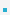5. DeclarationsAll variables must be declared before use, although certain declarations can be made implicitly by    content.The general format for a declaration is
type variable-name [=value].Examples:
 – char x; /* uninitialized */ – char x=’A’; /* initialized to ’A’*/ – char x=’A’, y=’B’; /*multiple variables initialized */ – char x=y=’Z’; /*multiple initializations */

Are the following declarations right?int x=017; int y=12;                    /* is x>y? */short int s=0xFFFF12;       /* correct? */char c=−1; unsigned char uc=−1; /* correct? */puts("hel"+"lo"); puts("hel""lo");      /* which is correct? */enum sz{S=0, L=3, XL};      /* what is the value of XL? */enum sz{S=0, L=−3, XL};   /* what is the value of XL? */

 이전페이지 / 6 / 다음페이지